Torsion-free linear connection

(diff) ← Older revision | Latest revision (diff) | Newer revision → (diff)

Definition

Symbol-free definition

A linear connection on a differential manifold is said to be torsion-free or symmetric if it satisfies the following equivalent conditions:

• Its torsion is zero
• Whenever two vector fields are such that their Lie bracket is zero, then the covariant derivative of either with respect to the other are equal.

Definition with symbols

A linear connection$\nabla$ on a differential manifold$M$ is said to be torsion-free or symmetric if it satisfies the following equivalent conditions:

• The torsion of$\nabla$ is a zero map, viz: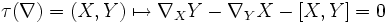$\tau(\nabla) = (X,Y) \mapsto \nabla_XY - \nabla_YX - [X,Y] = 0$

• Whenever$X$ and$Y$ are vector fields on an open subset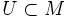$U \subset M$ such that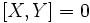$[X,Y]= 0$ on$U$, then: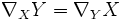$\nabla_X Y = \nabla_YX$

Definition in local coordinates

In local coordinates, a linear connection is torsion-free if the Christoffel symbols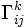$\Gamma_{ij}^k$ are symmetric in$i$ and$j$.

Facts

Set of all torsion-free linear connections

Further information: Affine space of torsion-free linear connections Recall that the set of all linear connections is an affine space, viz a translate of a linear subspace (the linear subspace being the maps that are tensorial in both variables).

The set of torsion-free linear connections is an affine subspace of this, in the sense that any affine combination of torsion-free linear connections is again a torsion-free linear connection.

The corresponding linear subspace for torsion-free linear connections are the symmetric 2-tensors.